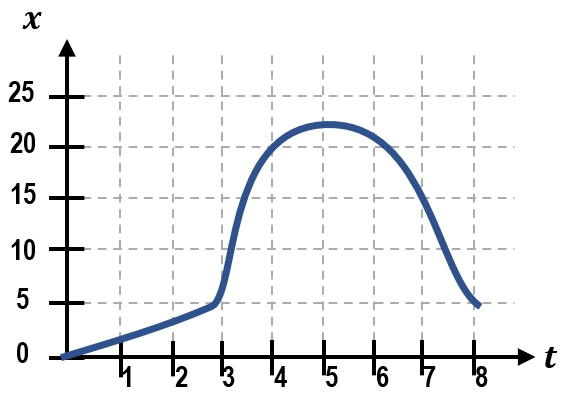Start typing, then use the up and down aroows to select an option from the list.
1.Problem

# The position-time graph for a ball on a track is shown below.(a) What is the ball’s velocity at 4s?(b) At what time(s) is the ball approximately travelling at -10m/s?(c) From t = 3 to 7s, what is the sign of the acceleration?Next question